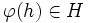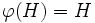# Injective endomorphism-invariant subgroup

(diff) ← Older revision | Latest revision (diff) | Newer revision → (diff)
BEWARE! This term is nonstandard and is being used locally within the wiki. [SHOW MORE]
This article defines a subgroup property: a property that can be evaluated to true/false given a group and a subgroup thereof, invariant under subgroup equivalence. View a complete list of subgroup properties[SHOW MORE]
If the ambient group is a finite group, this property is equivalent to the property: characteristic subgroup
View other properties finitarily equivalent to characteristic subgroup | View other variations of characteristic subgroup | Read a survey article on varying characteristic subgroup

## Definition

QUICK PHRASES: invariant under all injective endomorphisms, injective endomorphism-invariant

### Equivalent definitions in tabular format

Below are many equivalent definitions of injective endomorphism-invariant subgroup.

No. Shorthand A subgroup of a group is characteristic in it if... A subgroup$H$ of a group$G$ is called a characteristic subgroup of$G$ if ...
1 injective endomorphism-invariant every injective endomorphism of the whole group takes the subgroup to within itself for every injective endomorphism$\varphi$ of$G$,$\varphi(H) \subseteq H$. More explicitly, for any$h \in H$ and$\varphi \in \operatorname{End}(G)$ that is injective,$\varphi(h) \in H$
2 injective endomorphisms restrict to endomorphisms every injective endomorphism of the group restricts to an endomorphism of the subgroup. for every injective endomorphism$\varphi$ of$G$,$\varphi(H) \subseteq H$ and$\varphi$ restricts to an endomorphism of$H$
3 injective endomorphisms restrict to injective endomorphisms every injective endomorphism of the group restricts to an injective endomorphism of the subgroup for every injective endomorphism$\varphi$ of$G$,$\varphi(H) = H$ and$\varphi$ restricts to an injective endomorphism of$H$
This definition is presented using a tabular format. |View all pages with definitions in tabular format

## Formalisms

### Function restriction expression

This subgroup property is a function restriction-expressible subgroup property: it can be expressed by means of the function restriction formalism, viz there is a function restriction expression for it.
Find other function restriction-expressible subgroup properties | View the function restriction formalism chart for a graphic placement of this property
Function restriction expression$H$ is an injective endomorphism-invariant subgroup of$G$ if ... This means that injective endomorphism-invariance is ... Additional comments
injective endomorphism$\to$ function every injective endomorphism of$G$ sends every element of$H$ to within$H$ the invariance property for injective endomorphisms
injective endomorphism$\to$ endomorphism every injective endomorphism of$G$ restricts to an endomorphism of$H$ the endo-invariance property for injective endomorphisms; i.e., it is the invariance property for injective endomorphism, which is a property stronger than the property of being an endomorphism
injective endomorphism$\to$ injective endomorphism every injective endomorphism of$G$ restricts to a injective endomorphism of$H$ the balanced subgroup property for injective endomorphisms Hence, it is a t.i. subgroup property, both transitive and identity-true

## Relation with other properties

### Stronger properties

Property Meaning Proof of implication Proof of strictness (reverse implication failure) Intermediate notions

### Weaker properties

Property Meaning Proof of implication Proof of strictness (reverse implication failure) Intermediate notions
characteristic subgroup invariant under all automorphisms injective endomorphism-invariant implies characteristic characteristic not implies injective endomorphism-invariant |FULL LIST, MORE INFO
normal subgroup invariant under all inner automorphisms (via characteristic) (via characteristic) Characteristic subgroup|FULL LIST, MORE INFO

## Metaproperties

BEWARE! This section of the article uses terminology local to the wiki, possibly without giving a full explanation of the terminology used (though efforts have been made to clarify terminology as much as possible within the particular context)

Here is a summary:

Metaproperty name Satisfied? Proof Difficulty level (0-5) Statement with symbols
transitive subgroup property Yes follows from balanced implies transitive If$H \le K \le G$ are groups such that$H$ is injective endomorphism-invariant in$K$ and$K$ is injective endomorphism-invariant in$G$, then$H$ is injective endomorphism-invariant in$G$.
trim subgroup property Yes Obvious reasons 0 In any group$G$, the trivial subgroup$\{ e \}$ and the whole group$G$ are injective endomorphism-invariant in$G$
strongly intersection-closed subgroup property Yes follows from invariance implies strongly intersection-closed If$H_i, i \in I$, are all injective endomorphism-invariant in$G$, so is the intersection of subgroups$\bigcap_{i \in I} H_i$.
strongly join-closed subgroup property Yes follows from endo-invariance implies strongly join-closed If$H_i, i \in I$, are all injective endomorphism-invariant in$G$, so is the join of subgroups$\langle H_i \rangle_{i \in I}$